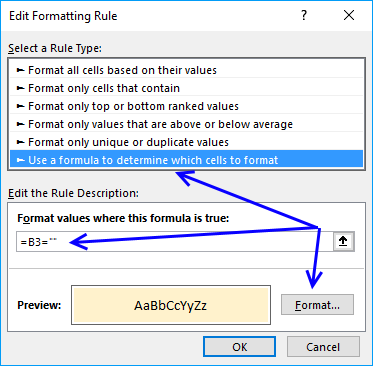Author: Oscar Cronquist Article last updated on January 14, 2019

The picture above shows you conditional formatting formula that highlights matching records based on criteria in row 3 and 4.

Conditional formatting formula:

=SUMPRODUCT(IF(AND(ISBLANK(\$B\$3:\$B\$4)), 1, (\$B\$3<=\$B7)*(\$B\$4>=\$B7))*(IF(ISBLANK(\$C\$3), 1, \$C\$3=\$C7))*(IF(ISBLANK(\$D\$3), 1, \$D\$3=\$D7))*(IF(AND(ISBLANK(\$E\$3:\$E\$4)), 1, (\$E\$3<=\$E7)*(\$E\$4>=\$E7))))

### How to apply conditional formatting formula

Make sure you adjust cell references to your excel sheet.

1. Select cells B7:E28
2. Click "Home" tab
3. Click "Conditional Formatting" button
4. Click "New Rule.."
5. Click "Use a formula to determine which cells to format"
6. Type the above formula in "Format values where this formula is TRUE" window.(The formula shown in the image above is not used in this article.)
7. Click "Format.." button
8. Click "Fill" tab
9. Select a color for highlighted cells.
10. Click "Ok"
11. Click "Ok"
12. Click "Ok"

### How the conditional formatting formula works in cell C8

Step 1 - Understand relative and absolute cell references

A cell reference may or may not have dollar signs in front of the reference, it indicates the cell reference is fixed meaning it won't change when the cell is copied to other cells.

Example, cell reference \$D3 is fixed to column D (absolute cell reference), however, row number 3 changes (relative cell reference) when the cell is copied to another cell.

Note, an absolute cell reference (fixed) changes if you insert/delete a row or column.

Step 2 - First criterion, dates

I have bolded the first date criteria below:

=SUMPRODUCT(IF(AND(ISBLANK(\$B\$3:\$B\$4)), 1, (\$B\$3<=\$B8)*(\$B\$4>=\$B8))*(IF(ISBLANK(\$C\$3), 1, \$C\$3=\$C8))*(IF(ISBLANK(\$D\$3), 1, \$D\$3=\$D8))*(IF(AND(ISBLANK(\$E\$3:\$E\$4)), 1, (\$E\$3<=\$E8)*(\$E\$4>=\$E8))))

IF(AND(ISBLANK(\$B\$3:\$B\$4)), 1, (\$B\$3<=\$B8)*(\$B\$4>=\$B8))

becomes

IF(AND(ISBLANK({40554;40568])), 1, (40554<=40574)*(40568>=40574))

becomes

IF(AND({FALSE;FALSE}), 1, (TRUE)*(FALSE))

becomes

IF(FALSE), 1, (TRUE)*(FALSE))

becomes

IF(FALSE), 1,0) returns 0.

Step 3 - Second criterion, color

I have bolded the second criterion below:

=SUMPRODUCT(IF(AND(ISBLANK(\$B\$3:\$B\$4)), 1, (\$B\$3<=\$B8)*(\$B\$4>=\$B8))*(IF(ISBLANK(\$C\$3), 1, \$C\$3=\$C8))*(IF(ISBLANK(\$D\$3), 1, \$D\$3=\$D8))*(IF(AND(ISBLANK(\$E\$3:\$E\$4)), 1, (\$E\$3<=\$E8)*(\$E\$4>=\$E8))))

IF(ISBLANK(\$C\$3), 1, \$C\$3=\$C8)

becomes

IF(ISBLANK("blue"), 1, "blue"="orange")

becomes

IF(FALSE, 1, False) returns FALSE.

Step 4 - Third criterion, products

I have bolded the third criterion below:

=SUMPRODUCT(IF(AND(ISBLANK(\$B\$3:\$B\$4)), 1, (\$B\$3<=\$B8)*(\$B\$4>=\$B8))*(IF(ISBLANK(\$C\$3), 1, \$C\$3=\$C8))*(IF(ISBLANK(\$D\$3), 1, \$D\$3=\$D8))*(IF(AND(ISBLANK(\$E\$3:\$E\$4)), 1, (\$E\$3<=\$E8)*(\$E\$4>=\$E8))))

IF(ISBLANK(\$D\$3), 1, \$D\$3=\$D8)

becomes

IF(ISBLANK("Product 4"), 1, "Product 4"="Product 3")

becomes

IF(FALSE, 1, FALSE) returns FALSE.

Step 5 - Fourth criterion, price

I have bolded the fourth criteria below:

=SUMPRODUCT(IF(AND(ISBLANK(\$B\$3:\$B\$4)), 1, (\$B\$3<=\$B8)*(\$B\$4>=\$B8))*(IF(ISBLANK(\$C\$3), 1, \$C\$3=\$C8))*(IF(ISBLANK(\$D\$3), 1, \$D\$3=\$D8))*(IF(AND(ISBLANK(\$E\$3:\$E\$4)), 1, (\$E\$3<=\$E8)*(\$E\$4>=\$E8))))

IF(AND(ISBLANK(\$E\$3:\$E\$4)), 1, (\$E\$3<=\$E8)*(\$E\$4>=\$E8))

becomes

IF(AND(ISBLANK({15;25})), 1, (15<=67,8)*(25>=67,8))

becomes

IF(AND({FALSE;FALSE}), 1, (TRUE)*(FALSE))

becomes

IF(FALSE, 1, 0) returns 0.

Step 6 - All criteria together

=SUMPRODUCT(IF(AND(ISBLANK(\$B\$3:\$B\$4)), 1, (\$B\$3<=\$B7)*(\$B\$4>=\$B7))*(IF(ISBLANK(\$C\$3), 1, \$C\$3=\$C7))*(IF(ISBLANK(\$D\$3), 1, \$D\$3=\$D7))*(IF(AND(ISBLANK(\$E\$3:\$E\$4)), 1, (\$E\$3<=\$E7)*(\$E\$4>=\$E7))))

becomes

=SUMPRODUCT(0*FALSE*FALSE*0)

becomes

=SUMPRODUCT(0) returns 0. Row 8 is not highlighted.

Note: Conditional formatting is volatile meaning when the worksheet is recalculated all conditionally formatted cells are recalculated, this may slow down your worksheet considerably if used to a large degree.

Final notes

The formula also works if you only have one criterion or two criteria.